# The volume of water gathered by a tilted paraboloid antenna

## Homework Statement

[/B]
The diameter and depth of an antenna that is
shaped like a paraboloid are ##d = 2.0m## and ##s = 0.5m## respectively. The antenna is set up so that its axis of symmetry is at an angle ##\theta = 30^{\circ}## from it's usual vertical orientation. How much can the antenna gather water in this position?

Hint: set up your coordinate axes so that the axis of symmetry of the paraboloid points in the z-direction and its vertex is at the origin. In this case you can assume that the equation of the paraboloid is of the form ##z=a(x^2 + y^2)## and that the surface of the water is going to settle on a plane ##z=bx+c##. Present the volume of the water as an integral in the xyz-coordinates, but do not try to calculate it by hand. Use Matlab to approximate the value with the command ##vpa(I)##, where ##I## is the asked integral.

Correct answer: ##25 \ liters \ = 25dm^3 = 25 \times 10^{-3}m^3##

## Homework Equations

This is going to turn into the evaluation of either a two- or a three-dimensional integral, so the volume is either given by:
\begin{equation}
V = \iint_R f(x,y) dA
\end{equation}
or
\begin{equation}
V = \iiint_T 1 \ dV
\end{equation}

## The Attempt at a Solution

Since the antenna is at ##30^{\circ}## from the vertical position, if we set the coordinate axes as instructed, the slope ##b## of the plane delimiting the volume of water from above ##z = bx + c## equals ##tan\theta = tan(30^{\circ}) = \frac{1}{\sqrt{3}}##.

Since the plane touches the edge of the antenna dish, looking at the diameter and depth of the antenna gives us ##z = 1/2## when ##x = 1 \implies 1/2 = \frac{1}{\sqrt{3}} (1) + c \iff c = 1/2 - 1/\sqrt{3}##.

Therefore the equation of the plane becomes
\begin{equation}
z = \frac{1}{\sqrt{3}}x + \frac{1}{2} - \frac{1}{\sqrt{3}}
\end{equation}

Below this plane the volume is delimited by the paraboloid surface ##z = a(x^2 + y^2)##, where ##a(x^2 + y^2)## is the square of the radius ##r## of the circle projected onto the xy-plane by the paraboloid. Now because of its rotational symmetry, when ##x = 1## and ##y=1##, again ##z=1/2##. Then since ##d = 2 \implies r=1## and
$$a(x^2 + y^2) = a(2) = 1 \iff a = \frac{1}{2}$$

Therefore the equation of the paraboloid is of the form
\begin{equation}
z = \frac{1}{2}(x^2 + y^2)
\end{equation}

Now to find out where these two surfaces intersect (to find out the projection of the volume onto the xy-plane) we set these two surfaces to be equal. Then
\begin{align*}
\frac{1}{\sqrt{3}}x + \frac{1}{2} - \frac{1}{\sqrt{3}} &= \frac{1}{2}(x^2 + y^2) \\
\iff \frac{2}{\sqrt{3}}x + 1 - \frac{2}{\sqrt{3}} &= (x^2 + y^2)\\
\iff x^2 - \frac{2}{\sqrt{3}}x + y^2 &= 1-\frac{2}{\sqrt{3}} || \text{ Complete the square: } + \frac{1}{3}\\
\iff x^2 - \frac{2}{\sqrt{3}}x + \frac{1}{3} + y^2 &= \frac{4}{3}-\frac{2}{\sqrt{3}}\\
\iff (x-\frac{1}{\sqrt{3}})^2 + y^2 &= \frac{4}{3}-\frac{2}{\sqrt{3}}\\
\end{align*}
This is a circle centered at ##(\frac{1}{\sqrt{3}},0)## whose radius is ##r_c = \sqrt{\frac{4}{3}-\frac{2}{\sqrt{3}}} \approx 0.42##.

Now to set up the integral. We need to solve for x in the equation of the circle if we want to establish the limits of integration:
\begin{equation*}
x = \pm \sqrt{\frac{4}{3} - \frac{2}{\sqrt{3}} - y^2} +\frac{1}{\sqrt{3}}
\end{equation*}
Therefore when ##y## goes from ##-\sqrt{\frac{4}{3}-\frac{2}{\sqrt{3}}}## to ##\sqrt{\frac{4}{3}-\frac{2}{\sqrt{3}}}##, ##x## goes from ##-\sqrt{\frac{4}{3} - \frac{2}{\sqrt{3}} - y^2} +\frac{1}{\sqrt{3}}## to ##\sqrt{\frac{4}{3} - \frac{2}{\sqrt{3}} - y^2} +\frac{1}{\sqrt{3}}##. Finally we integrate in the ##z##-direction, where z goes from ##\frac{1}{2}(x^2 + y^2)## to ##\frac{1}{\sqrt{3}}x + \frac{1}{2} - \frac{1}{\sqrt{3}}##.

This gives us the triple integral (f me...)
\begin{align*}
V
&=\int_{-\sqrt{\frac{4}{3}-\frac{2}{\sqrt{3}}}}^{\sqrt{\frac{4}{3}-\frac{2}{\sqrt{3}}}}
\int_{-\sqrt{\frac{4}{3} - \frac{2}{\sqrt{3}} - y^2} +\frac{1}{\sqrt{3}}}^{\sqrt{\frac{4}{3} - \frac{2}{\sqrt{3}} - y^2} +\frac{1}{\sqrt{3}}}
\int_{\frac{1}{2}(x^2 + y^2)}^{\frac{1}{\sqrt{3}}x + \frac{1}{2} - \frac{1}{\sqrt{3}}} 1 \ dzdxdy
\end{align*}

I am ##not## doing this by hand. Using the code below I get a complex answer:
Code:
%Calculating the volume of a puddle collected by a tilted paraboloid
%antenna
syms x y theta r z
f(x,y) = x/sqrt(3) + 1/2 - 1/sqrt(3);
g(x,y) = 1/2 * (x^2 + y^2);
format rat

%Innermost integral
a = int(1,z,1/2 * (x^2 + y^2),1/sqrt(3) + 1/2 - 1/sqrt(3));
%Second innermost integral
b = int(a,x,-sqrt(4/3 - 2/sqrt(3) - y^2),sqrt(4/3 - 2/sqrt(3) - y^2));

%Volume of the puddle
V0 = int(b,y,-sqrt(4/3-2/sqrt(3)),sqrt(4/3-2/sqrt(3)));
V = vpa(V0)
##V =
0.25553393767920640581899176181759 + 0.00000000000000000000000014120312922006717511927170824155i
##

The real part has promising-looking digits, except they're too large by a factor of 10. Any suggestions on what I'm doing wrong? Thanks.

Last edited:

I thought I'd include some pictures: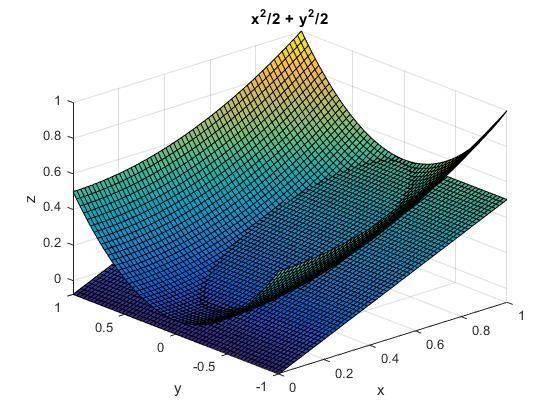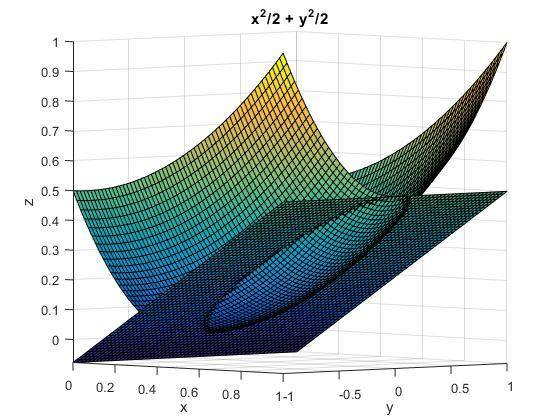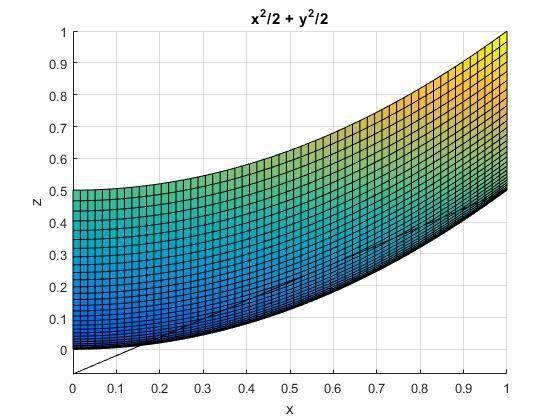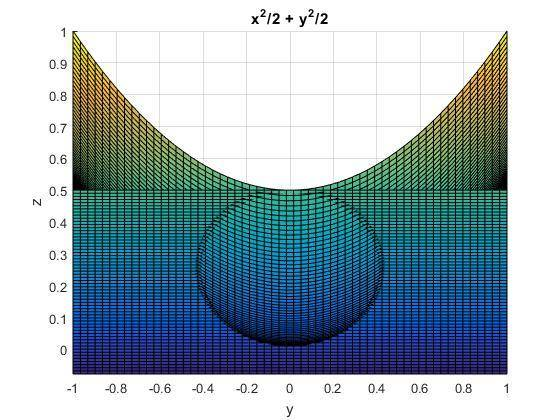SteamKing
Staff Emeritus
Homework Helper

## Homework Statement

[/B]
The diameter and depth of an antenna that is
shaped like a paraboloid are ##d = 2.0m## and ##s = 0.5m## respectively. The antenna is set up so that its axis of symmetry is at an angle ##\theta = 30^{\circ}## from it's usual vertical orientation. How much can the antenna gather water in this position?

Hint: set up your coordinate axes so that the axis of symmetry of the paraboloid points in the z-direction and its vertex is at the origin. In this case you can assume that the equation of the paraboloid is of the form ##z=a(x^2 + y^2)## and that the surface of the water is going to settle on a plane ##z=bx+c##. Present the volume of the water as an integral in the xyz-coordinates, but do not try to calculate it by hand. Use Matlab to approximate the value with the command ##vpa(I)##, where ##I## is the asked integral.

Correct answer: ##25 \ liters \ = 25dm^3 = 25 \times 10^{-3}m^3##

Since the OP talks about the diameter and depth of the antenna, I thought the shape should be more like this: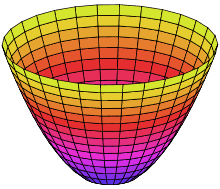But obviously shallower. It would be like having a large bowl tipped to one side and partially filled with water, like this: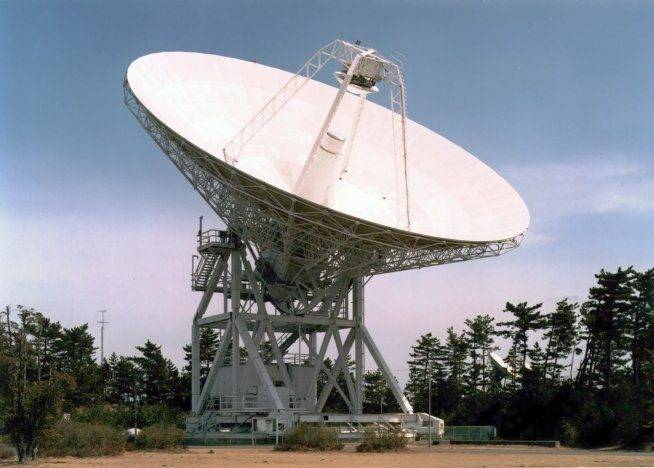I didn't get that from your pictures.

Since the OP talks about the diameter and depth of the antenna, I thought the shape should be more like this: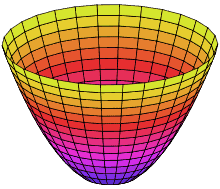But obviously shallower. It would be like having a large bowl tipped to one side and partially filled with water, like this:I didn't get that from your pictures.

Yeah, I sort of zoomed in on the intersection in the picture generated by Matlab, since it is tiny compared to the entire paraboloid. You wouldn't happen to know what I'm doing wrong, would you?SteamKing
Staff Emeritus
Homework Helper
Yeah, I sort of zoomed in on the intersection in the picture generated by Matlab, since it is tiny compared to the entire paraboloid. You wouldn't happen to know what I'm doing wrong, would you?It looked to me like your antenna was more in the shape of a parabolic cylinder than a paraboloid.

http://mathworld.wolfram.com/ParabolicCylinder.html

Even at the scale at which your pictures are drawn, the top of the antenna should definitely show a circular shape, since the diameter is 2.0 m, rather than the elongated shape which is actually depicted.

The fact that you integral is evaluating to a complex result also suggests something else is wrong in your derivation.

You can't just squint at the final result and pretend to see only the real part while ignoring the imaginary part.It looked to me like your antenna was more in the shape of a parabolic cylinder than a paraboloid.

http://mathworld.wolfram.com/ParabolicCylinder.html

Even at the scale at which your pictures are drawn, the top of the antenna should definitely show a circular shape, since the diameter is 2.0 m, rather than the elongated shape which is actually depicted.

The fact that you integral is evaluating to a complex result also suggests something else is wrong in your derivation.

You can't just squint at the final result and pretend to see only the real part while ignoring the imaginary part.My guess is the picture looks weird since I drew the surfaces using the ezsurf() -function. It plots surfaces into rectangular boxes, cutting their edges off. Getting back on point, I might have made a small mistake evaluating the value of the coefficient ##a##. The point (1,1) is not on the projection of the paraboloid. If I instead made things easy for myself and looked at point (1,0), ##a(x^2 + y^2) = a = 1## and if ##z_1=z_2##, then
\begin{align*}
\frac{1}{\sqrt{3}}x + \frac{1}{2} - \frac{1}{\sqrt{3}} &= x^2 + y^2\\
x^2 - \frac{1}{\sqrt{3}}x + y^2 &= \frac{1}{2} + \frac{1}{\sqrt{3}}|| + \frac{1}{4\times 3}\\
x^2 - \frac{1}{\sqrt{3}}x + \frac{1}{4\times 3} + y^2 &= \frac{1}{2} + \frac{1}{\sqrt{3}} + \frac{1}{4\times 3}||\text{ Complete the square}\\
(x-\frac{1}{2\sqrt{3}})^2 + y^2 &= \frac{7}{12} + \frac{1}{\sqrt{3}}
\end{align*}

This is a circle centered at ##(\frac{1}{2\sqrt{3}},0)## with a radius of ##r_c = \sqrt{\frac{7}{12} + \frac{1}{\sqrt{3}}}##. Again solving for x gives
$$x = \pm \sqrt{\frac{7}{12} - \frac{1}{\sqrt{3}}- y^2} + \frac{1}{2\sqrt{3}}$$

Then if y goes from ##-\sqrt{\frac{7}{12} + \frac{1}{\sqrt{3}}}## to ##\sqrt{\frac{7}{12} + \frac{1}{\sqrt{3}}}##, x goes from ##-\sqrt{\frac{7}{12} - \frac{1}{\sqrt{3}} - y^2} + \frac{1}{2\sqrt{3}}## to ##\sqrt{\frac{7}{12} - \frac{1}{\sqrt{3} }- y^2} + \frac{1}{2\sqrt{3}}## and z goes from ##x^2 + y^2## to ##\frac{1}{\sqrt{3}}x + \frac{1}{2} - \frac{1}{\sqrt{3}}##.

Then our integral becomes:
\begin{equation*}
V = \int_{-\sqrt{\frac{7}{12} + \frac{1}{\sqrt{3}}}}^{\sqrt{\frac{7}{12} + \frac{1}{\sqrt{3}}}}\int_{-\sqrt{\frac{7}{12} - \frac{1}{\sqrt{3}}- y^2} + \frac{1}{2\sqrt{3}}}^{\sqrt{\frac{7}{12} - \frac{1}{\sqrt{3}}- y^2} + \frac{1}{2\sqrt{3}}}\int_{x^2 + y^2}^{\frac{1}{\sqrt{3}}x + \frac{1}{2} - \frac{1}{\sqrt{3}}} \ 1 \ dzdxdy
\end{equation*}

Plugging this into matlab gives
##V =
0.000056229884935226496775211438294696 - 0.88438132601669853730091177717368i##. Still an imaginary number.LCKurtz
Homework Helper
Gold Member

## Homework Statement

[/B]

My guess is the picture looks weird since I drew the surfaces using the ezsurf() -function. It plots surfaces into rectangular boxes, cutting their edges off. Getting back on point, I might have made a small mistake evaluating the value of the coefficient ##a##. The point (1,1) is not on the projection of the paraboloid. If I instead made things easy for myself and looked at point (1,0), ##a(x^2 + y^2) = a = 1## and if ##z_1=z_2##, then$$\frac{1}{\sqrt{3}}x + \frac{1}{2} - \frac{1}{\sqrt{3}} = x^2 + y^2$$
The left side is ##z## on the plane, but isn't ##z## on the paraboloid ##z = \frac 1 2 (x^2+y^2)##?

Looks like my original derivation of ##a## was right, after all. Otherwise the paraboloid's height wouldn't be 0.5 at ##x = 1##. Here's a better picture from the side: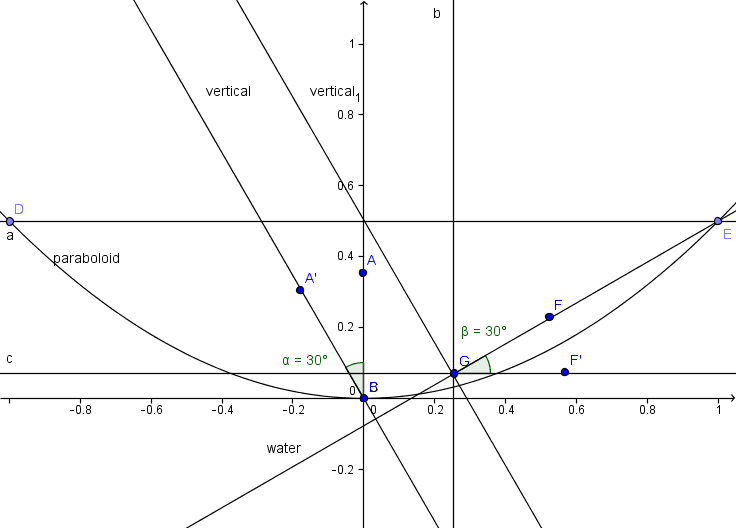I'm pretty sure the function for my water surface is correct, looking at this picture, and the equation for the paraboloid also seems to be correct. The angle ##\alpha## between the vertical and the axis of symmetry and ##\beta## should be equal, so my calculation of the slope should be correct as well. What the heck am I doing wrong?

Another picture from the direction of the axis of symmetry: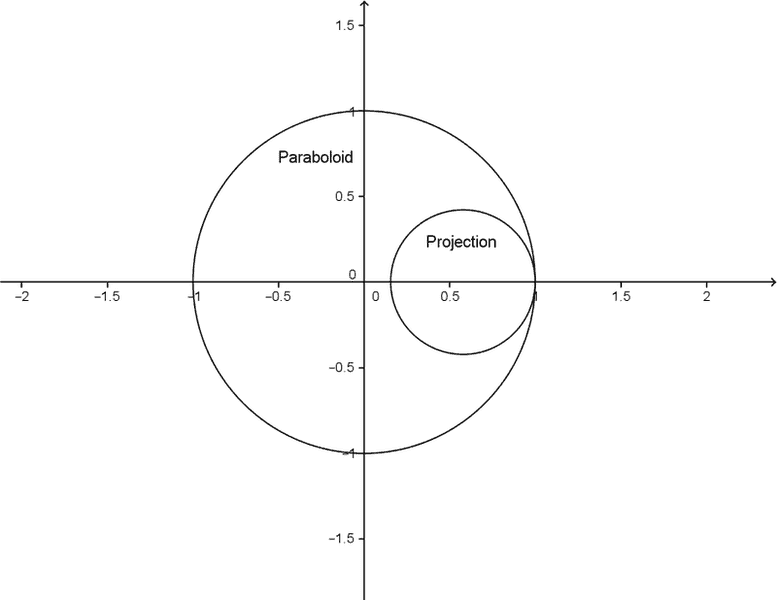It seems clear to me that the limits of integration should then be:

\begin{cases}
y: \pm \sqrt{\frac{4}{3} - \frac{2}{\sqrt{3}}}\\
x\text{ as a function of y}: \pm \sqrt{\frac{4}{3} - \frac{2}{\sqrt{3}}-y^2} + \frac{1}{\sqrt{3}}\\
z\text{ as a function of x and y}: \frac{1}{2}(x^2 + y^2) \text{ and } \frac{1}{\sqrt{3}} + \frac{1}{2} - \frac{1}{\sqrt{3}}
\end{cases}

My integral would then be
\begin{equation}
V = \int_{-\sqrt{\frac{4}{3} - \frac{2}{\sqrt{3}}}}^{\sqrt{\frac{4}{3} - \frac{2}{\sqrt{3}}}}\int_{-\sqrt{\frac{4}{3} - \frac{2}{\sqrt{3}}-y^2} + \frac{1}{\sqrt{3}}}^{\sqrt{\frac{4}{3} - \frac{2}{\sqrt{3}}-y^2} + \frac{1}{\sqrt{3}}}\int_{\frac{1}{2}(x^2 + y^2)}^{\frac{1}{\sqrt{3}}x + \frac{1}{2} - \frac{1}{\sqrt{3}}} 1 dzdxdy
\end{equation}
Using the following (fixed) code this time with all correct signs inside the radicals I get:
Code:
%Calculating the volume of a puddle collected by a tilted paraboloid
%antenna
syms x y theta r z
f(x,y) = x/sqrt(3) + 1/2 - 1/sqrt(3);
g(x,y) = 1/2*(x^2 + y^2);
format rat

%Innermost integral (z)
a = int(1,z,1/2*(x^2 + y^2),1/sqrt(3)*x + 1/2 - 1/sqrt(3));
%Second innermost integral(x)
b = int(a,x,-sqrt(4/3 - 2/sqrt(3) - y^2)+1/sqrt(3),sqrt(4/3 - 2/sqrt(3) - y^2)+1/sqrt(3));

%Volume of the puddle(integral in the y-direction)
V0 = int(b,y,-sqrt(4/3-2/sqrt(3)),sqrt(4/3-2/sqrt(3)));
V = vpa(V0)

V = 0.025061800479770940234396065694476 + 1.2255888711136160881691478299702e-34i

Then the real part of ##V\approx 0.025##, and since we used the units ##m^3## in our calculation, this is equal to ##25 \times 10^{-3}m^3 = 25dm^3 = 25 liters##, which is the correct answer.

Why is it including the imaginary part, though?

Last edited:
SteamKing
Staff Emeritus
Homework Helper
Another picture from the direction of the axis of symmetry:
View attachment 99592

It seems clear to me that the limits of integration should then be:

\begin{cases}
y: \pm \sqrt{\frac{4}{3} - \frac{2}{\sqrt{3}}}\\
x\text{ as a function of y}: \pm \sqrt{\frac{4}{3} - \frac{2}{\sqrt{3}}-y^2} + \frac{1}{\sqrt{3}}\\
z\text{ as a function of x and y}: \frac{1}{2}(x^2 + y^2) \text{ and } \frac{1}{\sqrt{3}} + \frac{1}{2} - \frac{1}{\sqrt{3}}
\end{cases}

My integral would then be
\begin{equation}
V = \int_{-\sqrt{\frac{4}{3} - \frac{2}{\sqrt{3}}}}^{\sqrt{\frac{4}{3} - \frac{2}{\sqrt{3}}}}\int_{-\sqrt{\frac{4}{3} - \frac{2}{\sqrt{3}}-y^2} + \frac{1}{\sqrt{3}}}^{\sqrt{\frac{4}{3} - \frac{2}{\sqrt{3}}-y^2} + \frac{1}{\sqrt{3}}}\int_{\frac{1}{2}(x^2 + y^2)}^{\frac{1}{\sqrt{3}}x + \frac{1}{2} - \frac{1}{\sqrt{3}}} 1 dzdxdy
\end{equation}
Using the following (fixed) code this time with all correct signs inside the radicals I get:
Code:
%Calculating the volume of a puddle collected by a tilted paraboloid
%antenna
syms x y theta r z
f(x,y) = x/sqrt(3) + 1/2 - 1/sqrt(3);
g(x,y) = 1/2*(x^2 + y^2);
format rat

%Innermost integral (z)
a = int(1,z,1/2*(x^2 + y^2),1/sqrt(3)*x + 1/2 - 1/sqrt(3));
%Second innermost integral(x)
b = int(a,x,-sqrt(4/3 - 2/sqrt(3) - y^2)+1/sqrt(3),sqrt(4/3 - 2/sqrt(3) - y^2)+1/sqrt(3));

%Volume of the puddle(integral in the y-direction)
V0 = int(b,y,-sqrt(4/3-2/sqrt(3)),sqrt(4/3-2/sqrt(3)));
V = vpa(V0)

V = 0.025061800479770940234396065694476 + 1.2255888711136160881691478299702e-34i

Then the real part of ##V\approx 0.025##, and since we used the units ##m^3## in our calculation, this is equal to ##25 \times 10^{-3}m^3 = 25dm^3 = 25 liters##, which is the correct answer.

Why is it including the imaginary part, though?
1.226 × 10-34 i ≈ 0

Maybe you don't need > 20 decimal places of precision on your calculations. 13 orders of magnitude is good enough to gauge the diameter of the orbit of Pluto to within about 1 meter.

1.226 × 10-34 i ≈ 0

Maybe you don't need > 20 decimal places of precision on your calculations. 13 orders of magnitude is good enough to gauge the diameter of the orbit of Pluto to within about 1 meter.

All I can say is... Lulz.

I guess I'm done, then.

Thank you and excelsior!